Updating search results...

# 32 Results

View
Selected filters:
• Number Line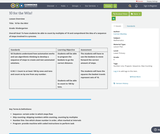Only Sharing Permitted
CC BY-NC-ND
Rating
0.0 stars

Subject:
Mathematics
Material Type:
Lesson Plan
Author:
Carmen Blackley
11/28/2017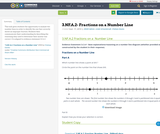Conditional Remix & Share Permitted
CC BY-NC-SA
Rating
0.0 stars

This task gives students the opportunity to analyze two number lines in order to identify the one that correctly shows an improper fraction. Students then communicate their understanding by describing the reasoning they used to determine their answer was correct. It is aligned to evidence statement 3.C.6-1

Subject:
Education
Mathematics
Material Type:
Activity/Lab
Assessment
Homework/Assignment
Author:
Linda Schoenbrodt
Chelsea Davies
09/17/2018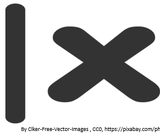Conditional Remix & Share Permitted
CC BY-NC
Rating
0.0 stars

In this seminar you will learn about the absolute value of numbers. You will learn how taking the absolute value affects both positive and negative numbers. You will use the techniques learned in this seminar to verify solutions to various other types of problems involving absolute value as you move forward. When looking at absolute value, you will identify how it can change a solution and the compare the difference that it makes in equations when there are multiple negative signs.StandardsCC.2.1.HS.F.2Apply properties of rational and irrational numbers to solve real world or mathematical problemsLearning TargetI can find the absolute value of a given number or numbers.Habits of MindPersistingCritical Thinking SkillAnalyze/evaluateAcademic/Concept VocabularyAbsolute valueNegativeNumber linePositive

Subject:
Mathematics
Algebra
Material Type:
Lesson Plan
Author:
Tracy Rains
03/19/2018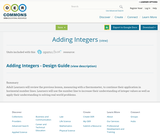Unrestricted Use
CC BY
Rating
0.0 stars

Adult Learners will review the previous lesson, measuring with a thermometer, to continue their application in horizontal number lines. Learners will use the number line to increase their understanding of integer values as well as apply their understanding to solving real world problems.

Subject:
Education
Mathematics
Material Type:
Interactive
Lesson Plan
Student Guide
Author:
Lisa Ramsey
05/14/2016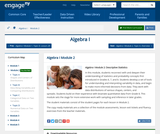Conditional Remix & Share Permitted
CC BY-NC-SA
Rating
0.0 stars

In this module, students reconnect with and deepen their understanding of statistics and probability concepts first introduced in Grades 6, 7, and 8. Students develop a set of tools for understanding and interpreting variability in data, and begin to make more informed decisions from data. They work with data distributions of various shapes, centers, and spreads. Students build on their experience with bivariate quantitative data from Grade 8. This module sets the stage for more extensive work with sampling and inference in later grades.

Subject:
Statistics and Probability
Material Type:
Module
Provider:
New York State Education Department
Provider Set:
EngageNY
08/01/2013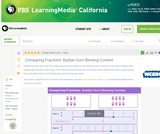Educational Use
Rating
0.0 stars

In this interactive activity adapted from Anneberg Learner&rsquo;s Teaching Math Grades 3&ndash;5, compare fractions on number lines to determine which class of students wins bubble-gum-blowing contests.

Subject:
Mathematics
Material Type:
Interactive
Provider:
PBS LearningMedia
Provider Set:
PBS Learning Media Common Core Collection
Author:
U.S. Department of Education
WGBH Educational Foundation
06/20/2012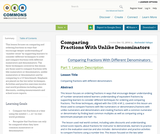Unrestricted Use
CC BY
Rating
0.0 stars

This lesson focuses on comparing and ordering fractions in ways that encourage deeper understanding of’ ‘number sense’ by supporting learners to consider different techniques to order and compare fractions with different numerators and denominators. The three techniques covered in this lesson are those used to compare fractions with like numerators or denominators, unlike numerators or denominators and by comparing to a 1/2 benchmark. Emphasis are placed on the two latter techniques. Activities and practice exercises involve real-world problems including sales discounts, cooking measurements and school score reports.

Subject:
Mathematics
Material Type:
Homework/Assignment
Lesson Plan
Author:
Rasheedat Yahaya
12/13/2016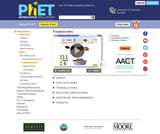Unrestricted Use
CC BY
Rating
0.0 stars

Explore fractions while you help yourself to 1/3 of a chocolate cake and wash it down with 1/2 a glass of orange juice! Create your own fractions using fun interactive objects. Match shapes and numbers to earn stars in the fractions games. Challenge yourself on any level you like. Try to collect lots of stars!

Subject:
Ratios and Proportions
Material Type:
Simulation
Provider:
Provider Set:
PhET Interactive Simulations
Author:
Ariel Paul
Kathy Perkins
Mike Dubson
Sam Reid
Trish Loeblein
08/17/2012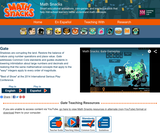Some Rights Reserved
Rating
0.0 stars

"Shadows are corrupting the land. Restore the balance of nature by exploring place value. Gate guides students in: lowering intimidation about large numbers and
decimals, understanding the meaning of place value, and realizing that the same mathematical concepts that apply to the ""easy"" integers apply to every order of magnitude."

Subject:
Mathematics
Material Type:
Game
Interactive
Provider:
Learning Games Lab
Author:
NMSU Learning Games Lab
07/20/2015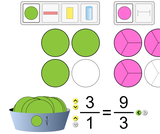Unrestricted Use
CC BY
Rating
0.0 stars

Build equivalent fractions with different denominators. Match shapes and numbers to earn stars in the game. Challenge yourself on any level you like. Try to collect lots of stars!

Subject:
Mathematics
Material Type:
Activity/Lab
Lesson
Lesson Plan
Simulation
Provider:
Provider Set:
PhET Interactive Simulations
Author:
Diana L Pez
Francisco Vilar Vasconcelos Raphael Alves Feitosa
Janelle Stanton
Leidiane Peixoto
Lilian Silva
Mait Vitorino E Nayara Voigt
Sherene Irvine
Trish Loeblein
03/12/2021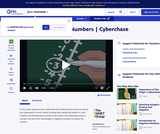Educational Use
Rating
4.0 stars

In this Cyberchase video segment, the CyberSquad locates the Cyberchase Council by using negative numbers.

Subject:
Mathematics
Material Type:
Lecture
Provider:
PBS LearningMedia
Provider Set:
PBS Learning Media: Multimedia Resources for the Classroom and Professional Development
Author:
U.S. Department of Education
WNET
07/24/2008Conditional Remix & Share Permitted
CC BY-NC
Rating
0.0 stars

Subject:
Mathematics
Material Type:
Full Course
Provider:
Pearson
10/06/2016Rating
0.0 stars

Equations and Inequalities

Type of Unit: Concept

Prior Knowledge

Students should be able to:

Add, subtract, multiply, and divide with whole numbers, fractions, and decimals.
Use the symbols <, >, and =.
Evaluate expressions for specific values of their variables.
Identify when two expressions are equivalent.
Simplify expressions using the distributive property and by combining like terms.
Use ratio and rate reasoning to solve real-world problems.
Order rational numbers.
Represent rational numbers on a number line.

Lesson Flow

In the exploratory lesson, students use a balance scale to find a counterfeit coin that weighs less than the genuine coins. Then continuing with a balance scale, students write mathematical equations and inequalities, identify numbers that are, or are not, solutions to an equation or an inequality, and learn how to use the addition and multiplication properties of equality to solve equations. Students then learn how to use equations to solve word problems, including word problems that can be solved by writing a proportion. Finally, students connect inequalities and their graphs to real-world situations.

Subject:
Mathematics
Algebra
Material Type:
Unit of Study
Provider:
Pearson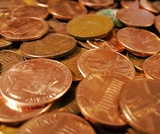Conditional Remix & Share Permitted
CC BY-NC
Rating
0.0 stars

Lesson OverviewStudents solve a classic puzzle about finding a counterfeit coin. The puzzle introduces students to the idea of a scale being balanced when the weight of the objects on both sides is the same and the scale being unbalanced when the objects on one side do not weigh the same as the objects on the other side.Key ConceptsThe concept of an inequality statement can be modeled using an unbalanced scale. The context—weighing a set of coins in order to identify the one coin that weighs less than the others—allows students to manipulate the weight on either side of the scale. In doing so, they are focused on the relationship between two weights—two quantities—and whether or not they are equal.Goals and Learning ObjectivesExplore a balance scale as a model for an equation or an inequality.Introduce formal meanings of equality and inequality.

Subject:
Numbers and Operations
Material Type:
Lesson Plan
Provider:
Pearson
09/21/2015Conditional Remix & Share Permitted
CC BY-NC
Rating
0.0 stars

Lesson OverviewStudents represent inequalities on a number line, find at least one value that makes the inequality true, and write the inequality using words.SWD:When calling on students, be sure to call on ELLs and to encourage them to actively participate. Understand that their pace might be slower or they might be shy or more reluctant to volunteer due to their weaker command of the language.SWD:Thinking aloud is one strategy for making learning visible. When teachers think aloud, they are externalizing their internal thought processes. Doing so may provide students with insights into mathematical thinking and ways of tackling problems. It also helps to model accurate mathematical language.Key ConceptsInequalities, like equations, have solutions. An arrow on the number line—pointing to the right for greater values and to the left for lesser values—can be used to show that there are infinitely many solutions to an inequality.The solutions to x < a are represented on the number line by an arrow pointing to the left from an open circle at a.Example: x < 2The solutions to x > a are represented on the number line with an arrow pointing to the right from an open circle at a.Example: x > 2The solutions to x ≤ a are represented on the number line with an arrow pointing to the left from a closed circle at a.Example: x ≤ 2The solutions to x ≥ a are represented on the number line with an arrow pointing to the right from a closed circle at a.Example: x ≥ 2Goals and Learning ObjectivesRepresent an inequality on a number line and using words.Understand that inequalities have infinitely many solutions.

Subject:
Numbers and Operations
Material Type:
Lesson Plan
Provider:
Pearson
09/21/2015Rating
0.0 stars

Getting Started

Type of Unit: Introduction

Prior Knowledge

Students should be able to:

Solve and write numerical equations for whole number addition, subtraction, multiplication, and division problems.
Use parentheses to evaluate numerical expressions.
Identify and use the properties of operations.

Lesson Flow

In this unit, students are introduced to the rituals and routines that build a successful classroom math community and they are introduced to the basic features of the digital course that they will use throughout the year.

An introductory card sort activity matches students with their partner for the week. Then over the course of the week, students learn about the lesson routines: Opening, Work Time, Ways of Thinking, Apply the Learning, Summary of the Math, and Reflection. Students learn how to present their work to the class, the importance of taking responsibility for their own learning, and how to effectively participate in the classroom math community.

Students then work on Gallery problems to further explore the program’s technology resources and tools and learn how to organize their work.

The mathematical work of the unit focuses on numerical expressions, including card sort activities in which students identify equivalent expressions and match an expression card to a word card that describes its meaning. Students use the properties of operations to identify equivalent expressions and to find unknown values in equations.

Subject:
Mathematics
Material Type:
Unit of Study
Provider:
PearsonConditional Remix & Share Permitted
CC BY-NC
Rating
0.0 stars

Gallery OverviewAllow students who have a clear understanding of the content thus far in the unit to work on Gallery problems of their choosing. You can then use this time to provide additional help to students who need review of the unit's concepts or to assist students who may have fallen behind on work.Gallery DescriptionRepresent a Math ProblemStudents explore the number line tool and the double number line tool. They use the number line tool to solve a problem about the weights of a cheetah and a fisher cat.Research ExpressionsStudents learn the difference between numerical expressions and variable expressions. They watch video tutorials, review worked examples, use the Glossary, and explore other resources.Fish TankStudents create diagrams and use text and images as they solve a problem about the size of a fish tank.

Subject:
Numbers and Operations
Material Type:
Lesson Plan
Provider:
Pearson
09/21/2015Rating
0.0 stars

Rational Numbers

Type of Unit: Concept

Prior Knowledge

Students should be able to:

Solve problems with positive rational numbers.
Plot positive rational numbers on a number line.
Understand the equal sign.
Use the greater than and less than symbols with positive numbers (not variables) and understand their relative positions on a number line.
Recognize the first quadrant of the coordinate plane.

Lesson Flow

The first part of this unit builds on the prerequisite skills needed to develop the concept of negative numbers, the opposites of numbers, and absolute value. The unit starts with a real-world application that uses negative numbers so that students understand the need for them. The unit then introduces the idea of the opposite of a number and its absolute value and compares the difference in the definitions. The number line and positions of numbers on the number line is at the heart of the unit, including comparing positions with less than or greater than symbols.

The second part of the unit deals with the coordinate plane and extends student knowledge to all four quadrants. Students graph geometric figures on the coordinate plane and do initial calculations of distances that are a straight line. Students conclude the unit by investigating the reflections of figures across the x- and y-axes on the coordinate plane.

Subject:
Mathematics
Numbers and Operations
Material Type:
Unit of Study
Provider:
PearsonConditional Remix & Share Permitted
CC BY-NC
Rating
0.0 stars

Students watch a video showing the highest and lowest locations on each of the continents. Then they create a diagram (a number line) for a book titled The World’s Highest and Lowest Locations. Students show four of the highest elevations and four of the lowest elevations in the world on their diagrams.Key ConceptsA complete number line has both positive numbers (to the right of 0) and negative numbers (to the left of 0).Negative numbers are written with a minus sign—for example, –12, which is pronounced “negative 12.”Positive numbers can be written with a plus sign for emphasis, such as +12, but a number without a sign, such as 12, is always interpreted as positive.Every number except 0 is either positive or negative. The number 0 is neither positive nor negative.Goals and Learning ObjectivesCreate a number line to show elevations that are both above and below sea level.

Subject:
Numbers and Operations
Material Type:
Lesson Plan
Provider:
Pearson
09/21/2015Conditional Remix & Share Permitted
CC BY-NC
Rating
0.0 stars

Students watch a dot get tossed from one number on a number line to the opposite of the number. Students predict where the dot will land each time based on its starting location.Key ConceptsThe opposite of a number is the same distance from 0 as the number itself, but on the other side of 0 on a number line.In the diagram, m is the opposite of n, and n is the opposite of m. The distance from m to 0 is d, and the distance from n to 0 is d; this distance to 0 is the same for both n and m. The absolute value of a number is its distance from 0 on a number line.Positive numbers are numbers that are greater than 0.Negative numbers are numbers that are less than 0.The opposite of a positive number is negative, and the opposite of a negative number is positive.Since the opposite of 0 is 0 (which is neither positive nor negative), then 0 = 0. The number 0 is the only number that is its own opposite.Whole numbers and the opposites of those numbers are all integers.Rational numbers are numbers that can be expressed as ab, where a and b are integers and b ≠ 0.Goals and Learning ObjectivesIdentify a number and its oppositeLocate the opposite of a number on a number lineDefine the opposite of a number, negative numbers, rational numbers, and integers

Subject:
Numbers and Operations
Material Type:
Lesson Plan
Provider:
Pearson